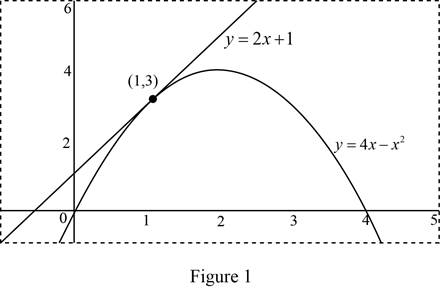# The slope of the tangent line to the parabola y = 4 x − x 2 at the point.### Single Variable Calculus: Concepts...

4th Edition
James Stewart
Publisher: Cengage Learning
ISBN: 9781337687805### Single Variable Calculus: Concepts...

4th Edition
James Stewart
Publisher: Cengage Learning
ISBN: 9781337687805

#### Solutions

Chapter 2.6, Problem 3E

(a)

To determine

## To find: The slope of the tangent line to the parabola y=4x−x2 at the point.

Expert Solution

The slope of the tangent line to the parabola y=4xx2 at the point (1,3),

(i) Using Definition 1 is m=2.

(ii) Using Equation 2 is m=2.

### Explanation of Solution

Given:

The slope of the tangent line to the parabola y=4xx2 at the point (1,3).

Formula used:

Definition 1: The slope of the tangent curve y=f(x) at the point P(a,f(a)), m=limxaf(x)f(a)xa.

Equation 2: The slope of the tangent line in definition 1 becomes, m=limh0f(a+h)f(a)h.

Section (i)

Obtain the slope of the tangent line to the parabola at the point (1, 3) by using Definition 1.

Substitute a=1 and f(a)=3 in Definition 1,

m=limx1(4xx2)3x1=limx14xx23x1=limx1(x24x+3)x1=limx1(x23xx+3)x1

The factors of (x24x+3) are (x3) and (x1).

Therefore, the slope of the tangent line to the parabola becomes,

m=limx1(x(x3)(x3))x1=limx1(x1)(x3)(x1)

Since the limit x approaches 1 but not equal to 1, cancel the common term (x1)(0) from both numerator and the denominator,

m=limx1((x3))=limx1(3x)=31=2

Thus, the slope of the tangent line to the parabola at the point (1, 3) is m=2_.

Section (ii)

Obtain the slope of the tangent line to the parabola at the point (1, 3) by using Equation 2.

Substitute a=1 and f(a)=3 in Equation 2,

m=limh0f(1+h)f(1)h=limh0(4(1+h)(1+h)2)3h=limh0(4+4h(1+h2+2h))3h=limh0(4+4h1h22h)3h

Simplify further and obtain the value of m.

m=limh0(3+2hh2)3h=limh03+2hh23h=limh02hh2h=limh0h(2h)h

Since the limit h tends to 0 but not equal to 0, cancel the common term h(0) from both the numerator and the denominator,

Thus, the slope of the tangent line becomes,

m=limh0(2h)=20=2

Thus, the slope of the tangent line to the parabola at the point (1, 3) by using equation 2 is m=2_.

(b)

To determine

### To find: The equation of the tangent line in part(a).

Expert Solution

The equation of the tangent line in part (a) is y=2x+1_.

### Explanation of Solution

Equation of the tangent line:

The equation of the tangent line to the curve y=f(x) at the point (a,f(a)) is,

yf(a)=f(a)(xa)

Since the tangent line to the curve y=f(x) at (a,f(a)) is the line through the point (a,f(a)) whose slope is equal to the derivative of a, the value of f(a)=2.

Substitute a=1, f(a)=3 and f(a)=2 in the equation of tangent line,

(yf(a))=f(a)(xa)y3=2(x1)=2x2

Isolate y as shown below.

y=2x2+3=2x+1

Thus, the equation of the tangent line is y=2x+1_.

(c)

To determine

Expert Solution

### Explanation of Solution

Calculation:

The equation of the tangent line is y=2x+1.

The equation of the given curve is y=4xx2.

Use the online graphing calculator to draw the graph of the functions as shown below in Figure 1.Graph:

Use the online graphing calculator to zoom toward the point (1,3) as shown below in Figure 2.From Figure 2, it is observed that when zoom in the graph toward the point (1, 3), the graph of the tangent line and the curve looks likes almost identical.

Hence, it is verified that the graph of the tangent line and the parabola zoom in toward the point (1, 3) until the tangent line are indistinguishable.

### Have a homework question?

Subscribe to bartleby learn! Ask subject matter experts 30 homework questions each month. Plus, you’ll have access to millions of step-by-step textbook answers!